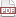Tip 43: Wrap substructures in parent structures

26 December 11. [link]PDF version

level: You have lots of structures floating around
purpose: quickly jump around your hierarchy of types

Part of a series of tips on POSIX and C. Start from the tip intro page, or get 21st Century C, the book based on this series.

As per tip 39, inheritance is obnoxiously simple in C: use substructures. If you have base_struct which is somehow fixed and immutable, but you want to add information to it, then set up a new struct with bonus features:

typedef struct {
base_struct base;
color_struct now_with_color;
audio_struct whizz, bang;
} better_struct;

OK, you're done: now when you allocate a better_struct, let us name it bb, you can use the original library of operations on bb.base as before.

What's the syntax for multiple inheritance and mixns? Add as many base structs to the new struct as you wish.

Apophenia does this: the apop_data struct is a gsl_matrix and a gsl_vector plus bells and whistles, which means that you can allocate an apop_data set ad and use the GNU Scientific Library's full arsenal on ad->matrix or ad->vector. That is, using functions that call the base struct is trivial.

The other direction can be an annoyance: you've got a gsl_matrix, and would like to use a function that requires a apop_data set with as little hassle as possible. How can you get the system to allocate the requisite extra stuff around the matrix that you care about?

Designated initializers, of course.

Apophenia has a wrapper to take dot products, because I hate the BLAS syntax (Basic Linear Algebra Subroutines--and for needs in the present day, is it ever basic).

#include <apop.h>

int main(){

//Let us allocate a matrix & vector via designated initializers.
//It's not easy: more on this below.
gsl_vector *v1 = &(gsl_vector){.size=3, .stride=1,
.data = (double[]){1, 2, 3}};
gsl_matrix *m1 = &(gsl_matrix){.size1=3, .size2=3, .tda=3,
.data = (double[]){1, 0, 0,
0, 0, 1,
0, 1, 0
}};

//wrap the matrix and vector in apop_data structs.
//This is easy.
printf("method 1:\n");
apop_data *d1 = &(apop_data){.matrix=m1};
apop_data *d2 = &(apop_data){.vector=v1};
apop_data *out = apop_dot(d1, d2);
apop_data_show(out);

//or even easier:
printf("method 2:\n");
out = apop_dot(
&(apop_data){.matrix=m1},
&(apop_data){.vector=v1});
apop_data_show(out);

//Or, via macros
#define Data_from_matrix(m) &(apop_data){.matrix=(m)}
#define Data_from_vector(v) &(apop_data){.vector=(v)}

out = apop_dot(Data_from_matrix(m1), Data_from_vector(v1));
printf("method 3:\n");
apop_data_show(out);
}

Making this happen actually took some planning, because there are now two ways to allocate the structure: you could use the official allocation function, or you can use designated initializers to pop a struct into existence. We generally don't like having two ways to do something, but for a struct explicitly designed to wrap an already-useful structure, it's hard to say no to such easy wrapping. Which is where the planning comes in: functions that take in the structure need to accept a fully-allocated data structure or a structure that is mostly empty. For the apop_data, it's not such a problem, because functions have an eye out for inputs which are mostly zero. The gsl_matrix and _vector, however, don't gracefully deal with having certain basically internal elements, the tda and stride, at zero.